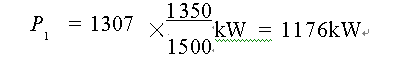﻿ 减速箱选型举例说明_减速箱原理知识_泰兴减速箱厂家

### 专业生产减速箱及配件德国技术，品质保证

#### 减速箱样本下载

SA     = 1. 5。选用功率 P2m   为:
P2       = ρ/η = 360 /0 . 98kW =  367. 35 kW
P2 m     = P2 KA SA     = 367. 35 ×1. 75 ×1 . 5kW  = 964. 3kW
i = 4. 5, 应当选 ZDY 系列某规格减速器。查表 10, 从接近要求转速的 n= 1500r / min 一档中 选取 P 1 ≥ P2m 的减速器, 试选 ZDY355, 该减速器在 i = 4. 5, n= 1500r / min 时, P 1 = 130kW, 当 n= 1350r / min 时折算公称功率 P 1   为:: P1      = 1176kW  > P2 m    =  964 . 3kW
ZDY355 -  4. 5 -  Ⅰ 的 P1   通过。若选取较小规格的 ZDY315 -  4 . 5 , 则 P1   通过。 第三步, 校核热功率 P G1   能否通过

P 2  / P1       = 360 /1176  = 0. 306  = 30. 6% , 查表 23, f3     = 1 . 25。P G1     = 425  > P2 t     = 424. 7kW
ZDY355 的 P G1   通过。

P ma x     = 760kW < 1. 8 P1     = 2352 kW

ZDY355 -  4. 5 -  Ⅰ

#### 相关文章